##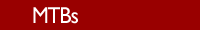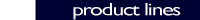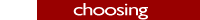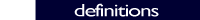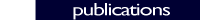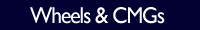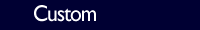### Choosing an MTB

Magnetic Torque Bars (MTBs) can be used to offset the average parasitic torques acting on a spacecraft. Common sources of parasitic torques are atmospheric drag, solar winds, gravity gradients, and magnetic dipole moments within the spacecraft. The parasitic torque acting on the spacecraft determines the torque required from the MTB. The torque generated by an MTB is given by the cross product of the MTB Moment vector and the Earth's Magnetic Field vector. Thus:

Torque = (MTB Moment) (Earth’s B-field) sin (theta)

where theta is the angle between the Earth’s B-field and the long axis of the MTB.

This equation indicates that torque cannot be produced about the length of the MTB or the axis of the Earth’s B-field. However, a spacecraft usually has 2 or 3 MTBs with unique orientations, so at a given instant in time torque can be generated in any direction except about the axis of the local Earth's magnetic field. As the satellite orbits around the earth, the direction of the Earth’s B-field changes relative to the satellite. This effect is called cross-orbit coupling. The cross-orbit coupling allows all axes of torque to be generated at some point in the orbit from only 2 MTBs, although it can be generated more efficiently from 3 MTBs. The sin (theta) factor reduces the moment being generated even with 2 or 3 MTBs. The moment required is given by:

Required Moment = (Average Parasitic Torque / Average B-field) x Angle Factor

where the angle factor is equal to 1/sin (theta). For a typical angle of 30 degrees the factor is 2.

The required moment calculated from the above equation is the minimum design moment. Round the required moment up to the nearest available design moment to determine the smallest suitable MTB. Cayuga Astronautics' MTB are guaranteed to produce the design moment at minimum bus voltage and room temperature. The satellite is assumed to operate near room temperature for most of its mission life. Guaranteeing operation at the minimum bus voltage provides a reasonable, but conservative design.

The MTB will produce more moment at the nominal bus voltage. The current produced at the nominal bus voltage can be estimated by dividing the nominal bus voltage by the nominal resistance. The nominal moment produced can be estimated by multiplying this current by the scale factor of the MTB. This moment should be compared to the linear moment and saturation moment to make sure that the core is still operating linearly and has not saturated. The moment at the nominal bus voltage can be used to choose the MTB for a less conservative design.

The MTB will produce less moment at a given supply voltage at the maximum ambient temperature. This is due to the increased resistance of the MTB’s copper windings at higher temperatures. The moment which can be guaranteed at all bus voltages and all ambient temperatures is the guaranteed moment. The guaranteed moment can be used to choose an MTB for a more conservative design.

### Example calculation

In Low Earth Orbit (LEO), the magnetic field from the Earth varies between 0.5 G (0.5 x 10^-4 T) near the poles and 0.2 G (0.2 x 10^-4 T) near the equator. If the parasitic torque is estimated to be 1 x 10^-3 N-m, then:

Required Moment = (Parasitic Torque / B Field) x Angle Factor

= (1 x 10^-3 N-m / (0.2 x 10^-4 T)) x 2

= 100 Am^2

The torque generated by the MTB is given by:

Torque = Moment x B-field x sin (theta)

At an angle of 30 degrees:

Torque = 100 Am^2 x 0.2 x 10^-4 T x 0.5

= 1 x 10^-3 N-m

Note that an 800 Am^2 MTB could produce a torque of 8 x 10^-3 N-m under the same conditions, comparable to a small reaction wheel. At a 90 degree angle and a 0.5 G field, the torque could be as large as 40 x 10^-3 N-m. In the graph below, the torque created by an 800 Am^2 MTB is plotted versus angle relative to the Earth's magnetic field. Click on the graph to enlarge.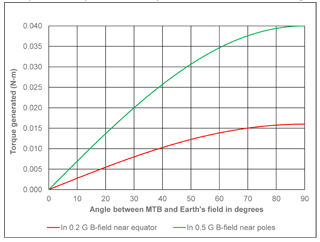### Disclaimer

The information on this page is meant to provide a simple method for sizing an MTB. In reality, one would need to integrate the parasitic torque acting on the spacecraft in all axes and come up with a complex activation schedule for all of the MTBs to counter the parasitic torque over a typical orbit.

In some cases, it may also be necessary to periodically change the attitude of the spacecraft prior to activating the MTBs, and dump the momentum stored in the onboard momentum storage devices (RWAs, Momentum Wheels or CMGs) when the spacecraft is in this “special momentum dumping attitude”.